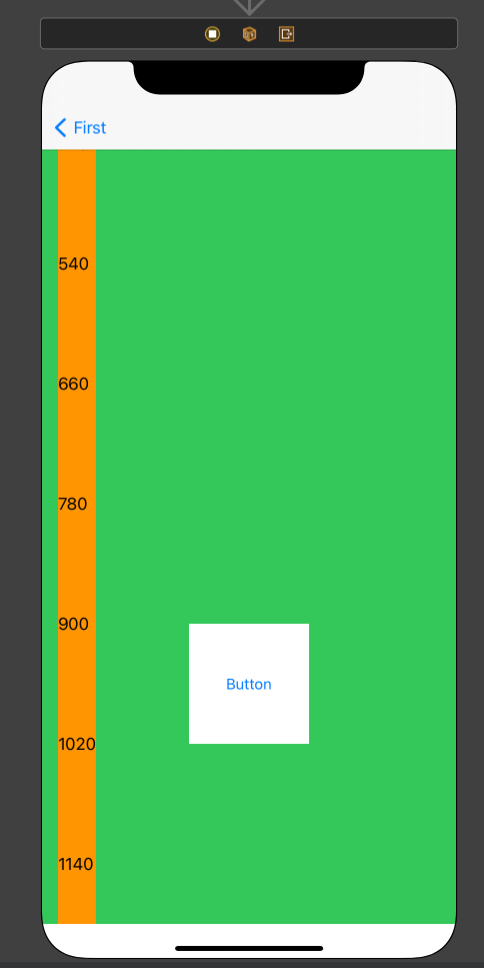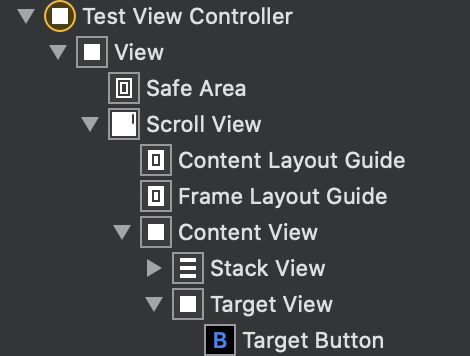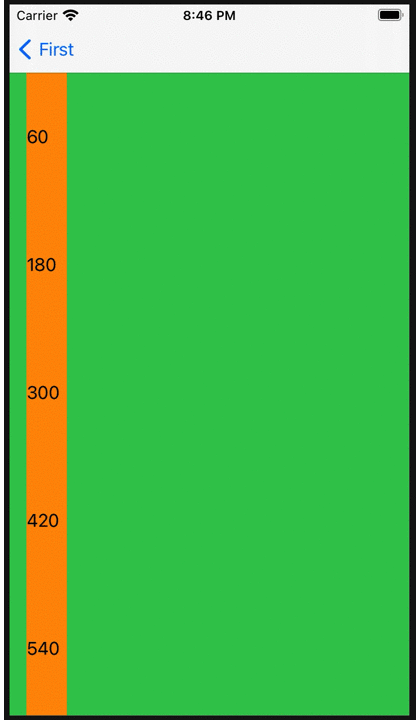# Now, I understand the coordinate transformation method of UIView (Swift)## Introduction

In rare cases, you may want to convert the coordinates using the convert method of `UIView`, but which one should you specify? I will forget it, so I will summarize it.

## View preparation

Let's perform coordinate conversion with the following configuration.The ScrollView is placed in the same size as the ViewController's View, and the `contentView` (green) is placed in it with the same width as the ScrollView and 1200 pt (StackView is for the leftmost memory). Inside the `contentView`, the 120 pt vertical and horizontal` targetView` (white) is placed at the top 900 pt of the `contentView`, and the horizontal is centered, and the button is placed in the center of the` targetView` (white).

## Coordinate transformation

The following method is provided in `UIView` for coordinate conversion, and it is converted using this method.

Both methods require the target View to be in the same Window. If the argument `view` is` nil`, it will be converted to the Window coordinate system. convert(_: to:) `point` specifies the coordinate system of the receiver, and` view` specifies the view of the coordinate system you want to convert.

``````func convert(_ point: CGPoint, to view: UIView?) -> CGPoint

// ex.
aView.convert(CGPoint.zero, to: bView)
``````

In the example, the upper left coordinate of `aView` is converted to the coordinate system of` bView`.

convert(_: from:) `point` specifies the coordinate system of` view` and converts it to the coordinate system of the receiver.

``````func convert(_ point: CGPoint, from view: UIView?) -> CGPoint

// ex.
aView.convert(CGPoint.zero, from: bView)
``````

In the example, the upper left coordinate of `bView` is converted to the coordinate system of` aView`.

## Practice

Try converting from the `targetView` coordinate system to the` scrollView` and ViewController `view` coordinate system when the button is pressed!

`````` @IBAction private func convertPoints(_ sender: Any) {
//Convert the upper right coordinates of targetView to the coordinate system of scrollView
let p1 = targetView.convert(CGPoint.zero, to: scrollView)
print("p1: \(p1)") // p1: (127.5, 900.0)

//Convert the upper right coordinates of targetView to the view coordinate system
let p2 = targetView.convert(CGPoint.zero, to: view)
print("p2: \(p2)") // p2: (127.5, 367.0) *Changes with offset of scrollView

//Convert the upper right coordinates of targetView to the coordinate system of scrollView
let p3 = scrollView.convert(CGPoint.zero, from: targetView)
print("p3: \(p3)") // p3: (127.5, 900.0)

//Convert the upper right coordinates of targetView to the view coordinate system
let p4 = view.convert(CGPoint.zero, from: targetView)
print("p4: \(p4)") // p4: (127.5, 367.0) *Changes with offset of scrollView
}
``````

As you can see from the above, p1 and p3, p2 and p4 have the same result.

Use this to adjust the scroll offset if `targetView` is off-screen when the button is pressed.

``````@IBAction private func convertPoints(_ sender: Any) {
//Convert the lower left coordinates of targetView to the view coordinate system
let p1 = targetView.convert(CGPoint(x: 0, y: targetView.frame.height), to: view)
//Check if the lower left of targetView is within the range of view
if !view.frame.contains(p1) {
//Scroll so that the targetView fits within the screen
let p2 = targetView.convert(CGPoint(x: 0, y: targetView.frame.height), to: scrollView)
scrollView.setContentOffset(.init(x: 0, y: p2.y - scrollView.frame.height), animated: true)
}
}
``````## in conclusion

If you think about the meaning of to and from, you can understand, but which coordinate system should `point` be attached to? I often get lost. .. ..

Don't get lost with this anymore! !! (Maybe: rolling_eyes :)

By the way, there is also a Rect conversion method.

I wonder if SwiftUI will not use this kind of thing: thinking: